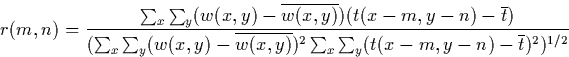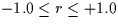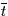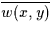Next: 5.3.1 Intermediate CROSS-CORR Output Up: 5 Raw Image Registration Previous: 5.2 General Method

# 5.3 Pattern Matching Algorithm - Step by Step

The following elaborates the procedures incorporated into CROSS-CORR. Fiducial locations which have been pre-defined for each camera and dispersion are accessed; these are hereafter referred to as the sampling locations. For each sampling location, the following steps are performed.

1.
The raw science image template is classified by its computed median Data Number (DN), and the ITF level possessing a comparable DN value at that location is chosen for correlation purposes.
2.
Any reseaux, hot spots, cosmic ray hits, and spectral information are ignored in the template during the correlation analysis. These artifacts contaminate the science template with features not present in the ITF window and are temporarily masked during the correlation procedure.

3.
The template is sequentially positioned throughout the window, and at each position a linear cross correlation is performed. For each position a correlation coefficient is computed and the corresponding coordinates (pixel accuracy only) are saved. By virtue of the sizes of the template and search windows, a total of forty-nine correlation coefficients are computed to determine the best pattern match location to pixel accuracy for each sampling location. These forty-nine coefficients are actually in the form of a 7 × 7 matrix which corresponds to the overlap positions of the template upon the window. The maximum coefficient in this sample is assumed to be the best representation for a true match.

The cross-correlation coefficient is defined as(1)
where. In this equation,is the average value of the template,is the average value of the portion of the window which is currently coincident with the template t(x,y), the line coordinate m = 0,1,2,...,(L-1), and the sample coordinate n = 0,1,2,...,(S-1). For the purposes of this implementation, L = 29 and S = 29 which are the nominal dimensions of the correlation window; the template dimensions are 23 × 23 pixels. Summations are performed over the coordinates common to both w and t.

4.
The maximum correlation coefficient must pass several statistical tests to be considered a valid correlation:
• There must be a minimum of 139 (13 × 13) non-rejected pixels in the template for the correlation to be performed at all (see Item 2);
• The maximum correlation coefficient cannot reside on the edge of the correlation matrix;
• The probability distribution criterion of less than 1% must be satisfied;
• There can be no other local'' maxima in the correlation matrix within 0.25 sigma of the absolute maximum coefficient. Sigma is the standard deviation of the correlation coefficient matrix.
• The maximum correlation coefficient must be at least two sigma greater than the rest of the coefficients in the matrix.

5.
If the coefficient is deemed valid, the position of the pattern match is then determined to sub-pixel accuracy. The original ITF window data is resampled into successively finer and finer sub-pixel increments and the cross correlation with the template is repeated until 0.125 sub-pixel accuracy is achieved.

If the coefficient is not validated by the tests described in Item 4, the sampling position is considered a bad location, and the algorithm passes onto the next sampling position.Next: 5.3.1 Intermediate CROSS-CORR Output Up: 5 Raw Image Registration Previous: 5.2 General Method
Karen Levay
12/4/1997